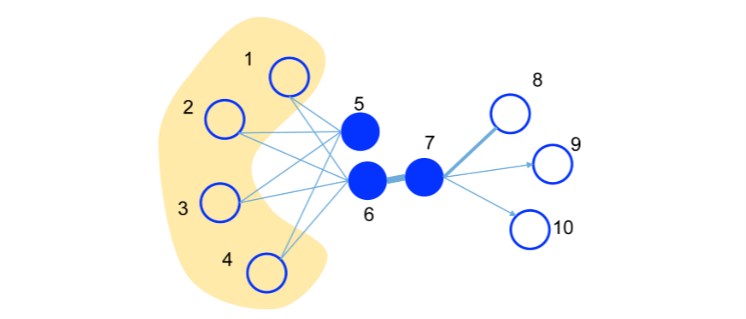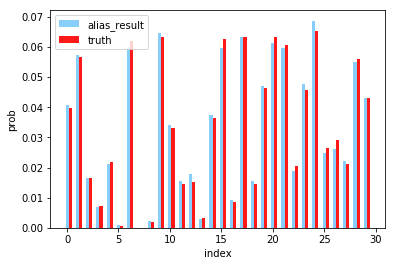DeepWalk：算法原理，实现和应用zhuanlan.zhihu.comLINE也是一种基于邻域相似假设的方法，只不过与DeepWalk使用DFS构造邻域不同的是，LINE可以看作是一种使用BFS构造邻域的算法。此外，LINE还可以应用在带权图中(DeepWalk仅能用于无权图)。

## first-order proximity

1阶相似度用于描述图中成对顶点之间的局部相似度，形式化描述为若,之间存在直连边，则边权即为两个顶点的相似度，若不存在直连边，则1阶相似度为0。 如上图，6和7之间存在直连边，且边权较大，则认为两者相似且1阶相似度较高，而5和6之间不存在直连边，则两者间1阶相似度为0。

## 1st-order为顶点的低维向量表示。（可以看作一个内积模型，计算两个item之间的匹配程度）是两个分布的距离，常用的衡量两个概率分布差异的指标为KL散度，使用KL散度并忽略常数项后有1st order 相似度只能用于无向图当中。

## Negative sampling是负边的个数。

## Edge Sampling

Alias Method:时间复杂度O(1)的离散采样方法zhuanlan.zhihu.com## 新加入顶点## 模型和损失函数定义

LINE使用梯度下降的方法进行优化，直接使用tensorflow进行实现，就可以不用人工写参数更新的逻辑了~

def line_loss(y_true, y_pred):
return -K.mean(K.log(K.sigmoid(y_true*y_pred)))

def create_model(numNodes, embedding_size, order='second'):

v_i = Input(shape=(1,))
v_j = Input(shape=(1,))

first_emb = Embedding(numNodes, embedding_size, name='first_emb')
second_emb = Embedding(numNodes, embedding_size, name='second_emb')
context_emb = Embedding(numNodes, embedding_size, name='context_emb')

v_i_emb = first_emb(v_i)
v_j_emb = first_emb(v_j)

v_i_emb_second = second_emb(v_i)
v_j_context_emb = context_emb(v_j)

first = Lambda(lambda x: tf.reduce_sum(
x*x, axis=-1, keep_dims=False), name='first_order')([v_i_emb, v_j_emb])
second = Lambda(lambda x: tf.reduce_sum(
x*x, axis=-1, keep_dims=False), name='second_order')([v_i_emb_second, v_j_context_emb])

if order == 'first':
output_list = [first]
elif order == 'second':
output_list = [second]
else:
output_list = [first, second]

model = Model(inputs=[v_i, v_j], outputs=output_list)

## 顶点负采样和边采样

def _gen_sampling_table(self):

# create sampling table for vertex
power = 0.75
numNodes = self.node_size
node_degree = np.zeros(numNodes)  # out degree
node2idx = self.node2idx

for edge in self.graph.edges():
node_degree[node2idx[edge]
] += self.graph[edge][edge].get('weight', 1.0)

total_sum = sum([math.pow(node_degree[i], power)
for i in range(numNodes)])
norm_prob = [float(math.pow(node_degree[j], power)) /
total_sum for j in range(numNodes)]

self.node_accept, self.node_alias = create_alias_table(norm_prob)

# create sampling table for edge
numEdges = self.graph.number_of_edges()
total_sum = sum([self.graph[edge][edge].get('weight', 1.0)
for edge in self.graph.edges()])
norm_prob = [self.graph[edge][edge].get('weight', 1.0) *
numEdges / total_sum for edge in self.graph.edges()]

self.edge_accept, self.edge_alias = create_alias_table(norm_prob)

## LINE应用

shenweichen/GraphEmbeddinggithub.comG = nx.read_edgelist('../data/wiki/Wiki_edgelist.txt',create_using=nx.DiGraph(),nodetype=None,data=[('weight',int)])

model = LINE(G,embedding_size=128,order='second')
model.train(batch_size=1024,epochs=50,verbose=2)
embeddings = model.get_embeddings()

evaluate_embeddings(embeddings)
plot_embeddings(embeddings)

micro-F1: 0.6403

macro-F1:0.5286